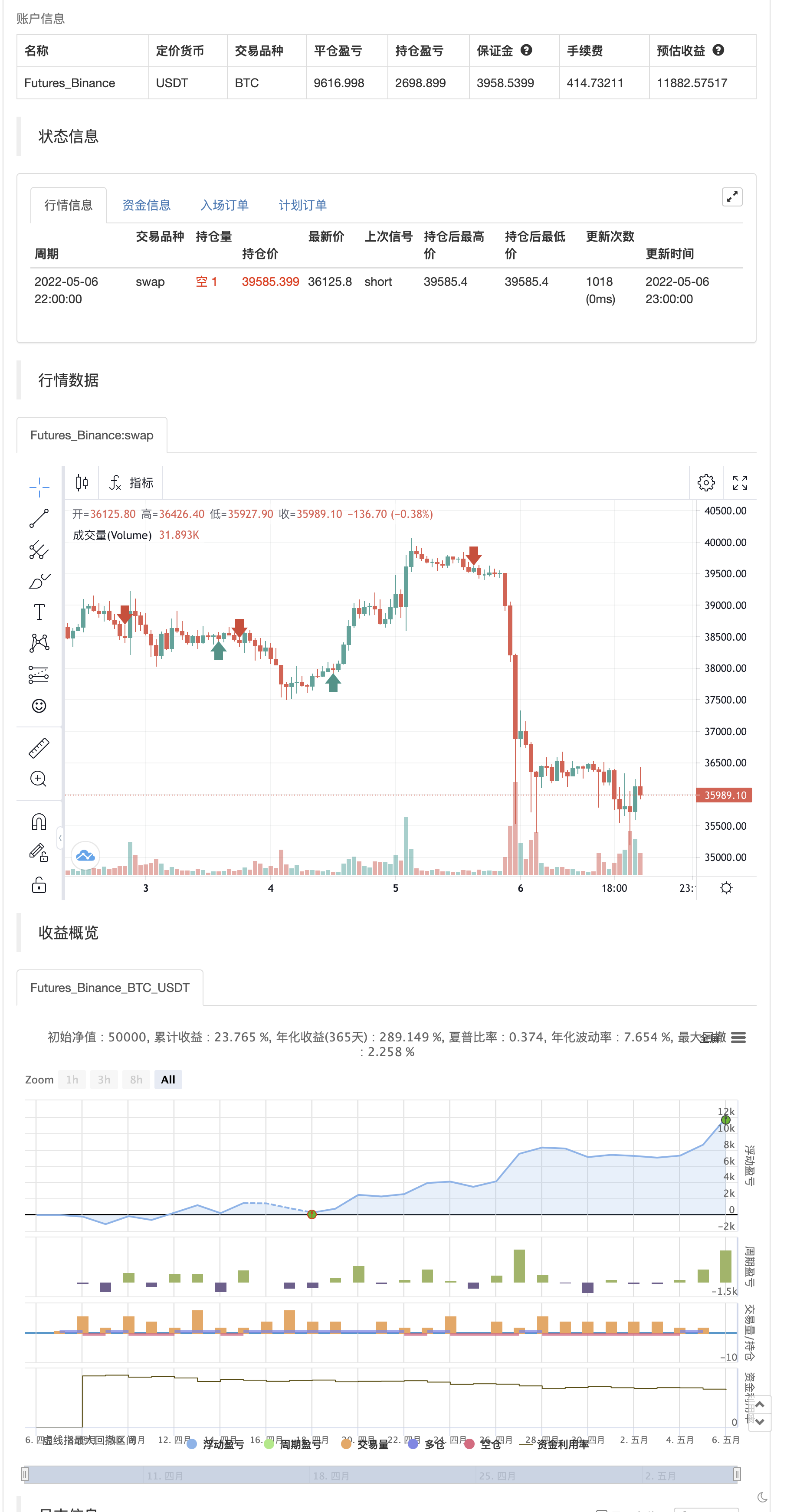# jma + dwma by multigrain

Author: 张超, Date: 2022-05-08 17:00:47
Tags: WMA

This crossover system was originally conceptualized by Jurik Research and made public to the world on their website.

The indicator consists of the faster Jurik Moving Average ( JMA ) and the slower Double Weighted Moving Average (DWMA). A long signal is shown when the JMA line crosses above the DWMA line (indicating a possible reversal in trend). A short signal is shown when the JMA line crosses below the DWMA line. Take profit signals are shown when the JMA line reverses directions. Alerts for signals are included in this indicator.

The default settings are not optimized for any timeframe. Both JMA and DWMA lines are defaulted to hidden.

Credit to @everget for the re-creation of the Jurik Moving Average in pinecsript.

backtest```/*backtest
start: 2022-04-07 00:00:00
end: 2022-05-06 23:59:00
period: 1h
basePeriod: 15m
exchanges: [{"eid":"Futures_Binance","currency":"BTC_USDT"}]
*/

// This source code is subject to the terms of the Mozilla Public License 2.0 at https://mozilla.org/MPL/2.0/
// @version=5

indicator('jma + dwma by multigrain', 'jma + dwma', overlay=true)

//NAME            TYPE               DEFVAL     TITLE               MIN     MAX         GROUP
longs           = input.bool        (true,      'Enable longs?')
shorts          = input.bool        (true,     'Enable shorts?')

jmaSrc          = input.source      (close,     'JMA Source',                           group='JMA')
jmaLen          = input.int         (7,         'JMA Length',       0,      100,        group='JMA')
jmaPhs          = input.int         (50,        'JMA Phase',        -100,   100,        group='JMA')
jmaPwr          = input.float       (1,         'JMA Power',        0.1,                group='JMA')

dwmaSrc         = input.source      (close,     'DWMA Source',                          group='DWMA')
dwmaLen         = input.int         (10,        'DWMA Length',      1,      100,        group='DWMA')

// Jurik Moving Average
f_jma(_src, _length, _phase, _power) =>
phaseRatio  = _phase < -100 ? 0.5 : _phase > 100 ? 2.5 : _phase / 100 + 1.5
beta        = 0.45 * (_length - 1) / (0.45 * (_length - 1) + 2)
alpha       = math.pow(beta, _power)
jma         = 0.0
e0          = 0.0
e0          := (1 - alpha) * _src + alpha * nz(e0)
e1          = 0.0
e1          := (_src - e0) * (1 - beta) + beta * nz(e1)
e2          = 0.0
e2          := (e0 + phaseRatio * e1 - nz(jma)) * math.pow(1 - alpha, 2) + math.pow(alpha, 2) * nz(e2)
jma         := e2 + nz(jma)
jma

// Double Weighted Moving Average
f_dwma(_src, _length) =>
ta.wma(ta.wma(_src, _length), _length)

// Calculations
jma             = f_jma             (jmaSrc,    jmaLen,     jmaPhs,     jmaPwr)
dwma            = f_dwma            (dwmaSrc,   dwmaLen)
long            = ta.crossover      (jma,       dwma)
long_tp         = ta.pivothigh      (jma,       1,          1)              and jma > dwma
short_tp        = ta.pivotlow       (jma,       1,          1)              and jma < dwma
short           = ta.crossunder     (jma,       dwma)
if longs